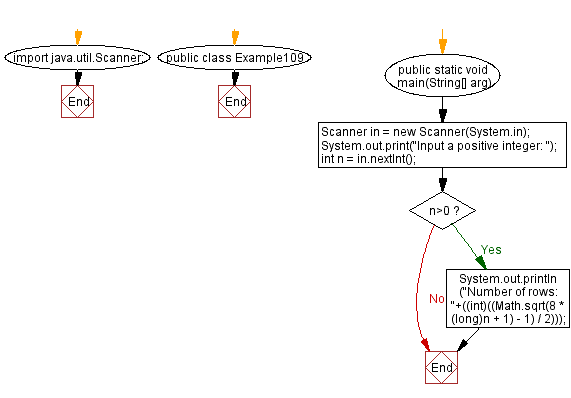﻿ Java: Make a staircase of n coins with k coins in every row# Java Exercises: Form a staircase shape of n coins where every k-th row must have exactly k coins

## Java Basic: Exercise-109 with Solution

Write a Java program to form a staircase shape of n coins where every k-th row must have exactly k coins.

Example 1:
n = 3
The coins can form the following rows:
\$
\$ \$
We will return 2 rows.
Example 2:
n = 4
The coins can form the following rows:
\$
\$ \$
\$
We will return 2 rows as the 3rd row is incomplete.
Example 3:
n = 5
The coins can form the following rows:
\$
\$ \$
\$ \$
We will return 2 rows as the 3rd row is incomplete.

Sample Solution:

Java Code:

``````import java.util.Scanner;
public class Example109 {
public static void main(String[] arg) {
Scanner in = new Scanner(System.in);
System.out.print("Input a positive integer: ");
int n = in.nextInt();
if (n>0)
{
System.out.println("Number of rows: "+((int)((Math.sqrt(8 * (long)n + 1) - 1) / 2)));
}
}
}
```
```

Sample Output:

```Input a positive integer: 5
Number of rows: 2
```

Flowchart:Java Code Editor:

What is the difficulty level of this exercise?

Test your Programming skills with w3resource's quiz.

﻿

## Java: Tips of the Day

getEnumMap

Converts to enum to Map where key is the name and value is Enum itself.

```public static <E extends Enum<E>> Map<String, E> getEnumMap(final Class<E> enumClass) {
return Arrays.stream(enumClass.getEnumConstants())
.collect(Collectors.toMap(Enum::name, Function.identity()));
}
```

Ref: https://bit.ly/3xXcFZt